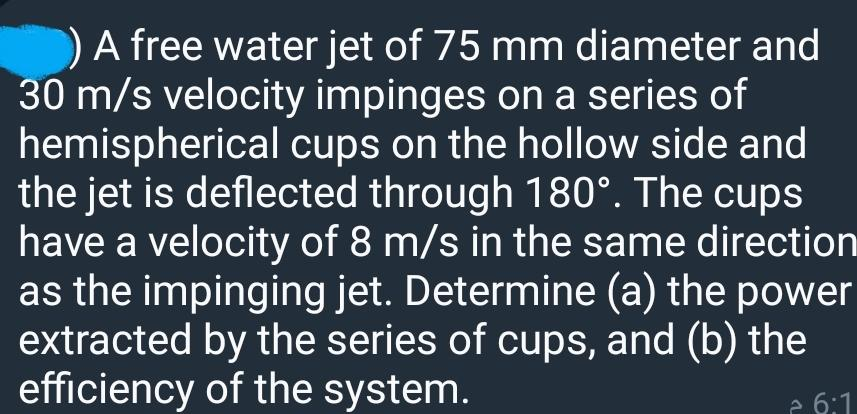# Question Solved1 AnswerAnswer me A free water jet of 75 mm diameter and 30 m/s velocity impinges on a series of hemispherical cups on the hollow side and the jet is deflected through 180°. The cups have a velocity of 8 m/s in the same direction as the impinging jet. Determine (a) the power extracted by the series of cups, and (b) the efficiency of the system. ? 6:1LXXLXO The Asker · Mechanical EngineeringAnswer me

Transcribed Image Text: A free water jet of 75 mm diameter and 30 m/s velocity impinges on a series of hemispherical cups on the hollow side and the jet is deflected through 180°. The cups have a velocity of 8 m/s in the same direction as the impinging jet. Determine (a) the power extracted by the series of cups, and (b) the efficiency of the system. ? 6:1
More
Transcribed Image Text: A free water jet of 75 mm diameter and 30 m/s velocity impinges on a series of hemispherical cups on the hollow side and the jet is deflected through 180°. The cups have a velocity of 8 m/s in the same direction as the impinging jet. Determine (a) the power extracted by the series of cups, and (b) the efficiency of the system. ? 6:1